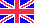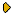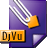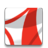Журнал Экспериментальной и Теоретической Физики
 НАЧАЛО | ПОИСК | ДЛЯ АВТОРОВ | ПОМОЩЬОбщая информация о журналеЗолотые страницыАдреса редакцииСодержание журналаСообщения редакцииПравила для авторовЗагрузить статьюПроверить статус статьиЖЭТФ, Том 125, Вып. 2, стр. 414 (Февраль 2004)
(Английский перевод - JETP, Vol. 98, No 2, p. 366, February 2004 доступен on-line на www.springer.com )

HEAT CAPACITY OF ISOLATED CLUSTERS

Поступила в редакцию: 10 Июня 2003

PACS: 61.20.Gy, 61.25.Bi, 61.43.Fs, 64.70.DvDJVU (95.5K)PDF (242.3K)

The character of interaction between the thermal (vibrational) and configurational cluster excitations is considered under adiabatic conditions when a cluster is a member of a microcanonical ensemble. A hiearchy of equilibration times determines the character of atomic equilibrium in the cluster. The behavior of atoms in the cluster can be characterized by two effective (mean) temperatures, corresponding to the solid and liquid aggregate states because a typical time for equilibration of atomic motion is less than the time of transition between the aggregate states. If the cluster is considered for a time much longer than the typical dwell time in either phase, then it is convenient to characterize the system by only one temperature, which is determined from the statistical-thermodynamical long-time average. These three temperatures are not far apart, nor are the cluster heat capacities evaluated on the basis of these definitions of temperature. The heat capacity of a microcanonical ensemble may be negative under some circumstances in a region of coexistence of two phases, provided the mean temperature is defined in terms of the mean kinetic energy, not as a the derivative of the energy with respect to the microcanonical entropy. However, we show that if the configurational excitation energy is small relative to the total excitation energy separating the phases, then, within the model of two aggregate states, the heat capacity is positive whichever definition is used for temperature. In addition, for a sufficiently large cluster, the maximum values of the microcanonical and canonical heat capacities are the same.

Сообщить о технических проблемах